# High School Math : Understanding 30-60-90 Triangles

## Example Questions

### Example Question #6 : Graphing The Sine And Cosine Functions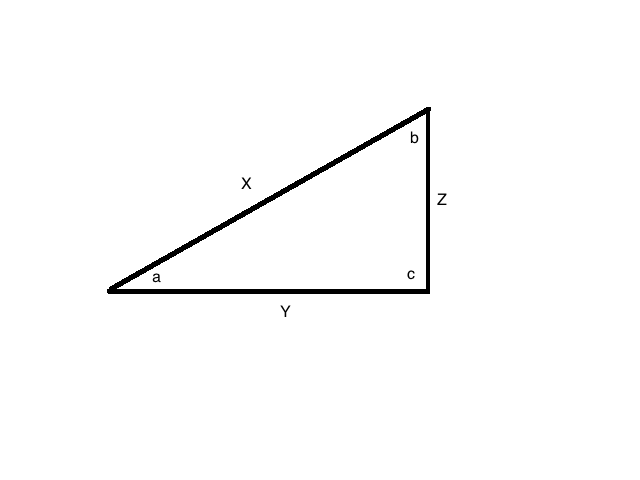In this figure,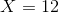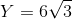, and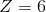. What is the value of angle?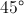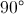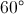Undefined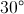Explanation:

Notice that these sides fit the pattern of a 30:60:90 right triangle: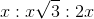.

In this case,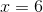.

Since angleis opposite, it must be.

### Example Question #7 : Graphing The Sine And Cosine Functions

A triangle has angles of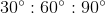. If the side opposite theangle is, what is the length of the side opposite?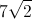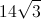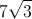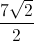The pattern foris that the sides will be.
If the side oppositeis, then the side oppositewill be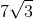.